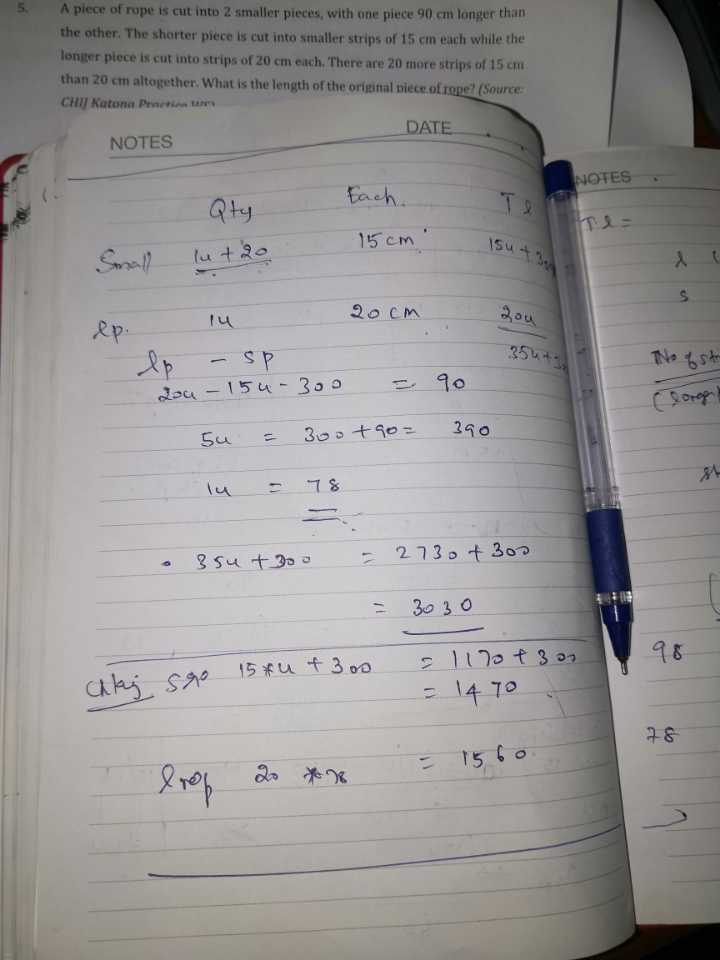# Question

A piece of rope is cut into two smaller pieces, with one piece 90 cm longer than the other . The shorter piece is cut into strips of 20 cm each. The are 20 more strips of 15 cm than20 cm altogether. What is the length of the original piece of rope ?

length of shorter pieces : 1u
number of shorter pieces : 1p
total length of longer pieces : (1u + 90)
number of longer pieces : (1p + 20)
1u ——-> 1p x 20 = 20p
(1u + 90) ——-> (1p + 20) x 15 = 15p + 300
20p + 90 = 15p + 300
1p = (300 – 90)/(20 – 15) = 42
42 x 20 + (42 + 20) x 15 = 1770

Ans : 1770 cm.

0 Replies 0 LikesEach short piece is 15 cm length. We tried your method and got solution

0 Replies 0 Likes